# Complete Feature Selection Techniques 4 - 2 Correlation Analysis

Summarize math intuition and demonstrate Correlation, Multicollinearity and Exploratory Factor Analysis for feature selection

Complete Feature Selection Techniques

1. Statistical Test & Analysis
2. Correlation Analysis
3. Dimension Reduction
4. Model Driven

# Correlation and Causation

Correlation is a measure of relationship between the values of two variables, if the relationship can be approximated by a line, then it is linear correlation and it can be presented in a scatter plot.

For example, x and y are two variables and their scatter plot as below

## Correlation does not imply Causation

If two variables have strong correlation, it only indicate the two variables tend to change together(positive or negative), but it can’t prove one variable change causes the change of the other variable. Below is an example

# Correlation Coefficient Definition

Following are three common(in feature selection) correlation coefficients which can quantitatively measure the degree of correlation.

## Pearson Correlation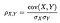source from wikipedia

Pearson correlation is focus on measuring how well the two variables following linear relationship

## Spearman’s Rank-Order Correlation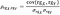source from wikipedia

rgX, rgY is rank order number for X and Y.

Rank order number can be

1. Ordinal Categorical Encoding on variable
2. Bucket or Discrete continuous variable into ordinal number

Compare with Pearson correlation, Spearman correlation is insensitive to outliers in variable because it uses ordinal rank order instead of original variable value.

## Kendall Rank Correlation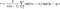source from wikipedia

Kendall Rank is generalized from Spearman correlation, it is more suitable to measure monotonic relationship of two variables.

## Significance Test

Once correlation coefficient is calculated, in order to verify the calculated coefficient is significantly different(a.k.a far away) from 0, we may need apply significance test with coefficient=0 as null hypothesis, please reference wikipedia for detailed test setup.

## Coefficients Interpretation

All the above correlation coefficients have range from -1 to +1, and the coefficient value indicates the degree of correlation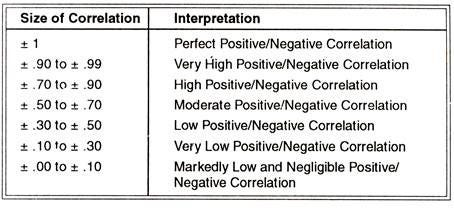Coefficients Interpretation from Correlation: Meaning, Types and Its Computation | Statistics

In feature selection scenario, we can keep the independent variables which have strong correlation with target variable, and the very low correlation variables are the candidates to be removed.

## Python Example

The example calculates correlation values between column TAX and RAD in Boston House data

`import numpy as npimport pandas as pd  from sklearn.datasets import load_bostonfrom scipy.stats import pearsonrfrom scipy.stats import spearmanrfrom scipy.stats import kendalltau%matplotlib inlineboston_dataset = load_boston()boston = pd.DataFrame(    boston_dataset.data,     columns=boston_dataset.feature_names)coef_pearsonr, p_pearsonr = pearsonr(boston['TAX'], boston['RAD'])coef_spearman, p_spearman = spearmanr(boston['TAX'], boston['RAD'])coef_Kendall, p_Kendall = kendalltau(boston['TAX'], boston['RAD'])corr_data = pd.DataFrame(columns=['Correlation','Pearson','Spearman','Kendall'])corr_data['Correlation'] = ['Coefficient','P-Value']corr_data['Pearson'] = [coef_pearsonr, p_pearsonr]corr_data['Spearman'] = [coef_spearman, p_spearman]corr_data['Kendall'] = [coef_Kendall, p_Kendall]corr_data.set_index(['Correlation'])`

# Multicollinearity and Regression Analysis

In a multiple regression, Multicollinearity means the existence of a high degree of correlation among the independent variables.

During regression process, the high correlation within independent variables will increase Coefficient Standard Error(SE), because

1. Correlated variables are not independent
2. Sampling bias in one individual variable will impact other correlated variables
3. This each other impact results in larger sampling bias for all the correlated variables.

Due to the increasing coefficient standard error

1. Coefficient t-statistic and p-value will become unreliable, so we are not confident to interpret prediction contribution for each individual variable via its coefficient.
2. Regression model will be sensitive to training data, different training data may generate regression model with big different coefficients

## Detection of Multicollinearity

Practically there are two ways to quickly detect the Multicollinearity in regression process

Correlation Matrix - Calculate correlation value for each pair of independent variables and verify the degree of correlation by reference the above coefficients interpretation table.

`import numpy as npimport matplotlib.pyplot as plt import pandas as pd  import seaborn as sns from sklearn.datasets import load_boston%matplotlib inlineboston_dataset = load_boston()boston = pd.DataFrame(boston_dataset.data, columns=boston_dataset.feature_names)correlation_matrix = boston.corr().round(2)plt.figure(figsize = (16,6))sns.heatmap(data=correlation_matrix, annot=True)`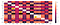|value|>0.7 identify high correlation feature pair

Variance Inflation Factors(VIF) - VIF quantifies the severity of multicollinearity for independent variables, it can discover the correlation among more than two variables. The process is below

Consider the following linear model with k independent variables

For each independent variable Xi, the Variance Inflation Factors VIFis

1. Build an OLS(least square linear regression) model Xi = OLS( Xj i ), which use Xi as target value and all other independent variables Xj i as input values
2. Calculate the OLS model R-Square Rᵢ², and VIF factor for variable Xi is

The general rule for interpreting VIF value is

1 = Not Correlated
Between 1 and 5 = Moderately Correlated
Greater than 5 = Highly Correlated

`from statsmodels.stats.outliers_influence import variance_inflation_factorvif_data = pd.DataFrame() vif_data["Feature"] = boston_dataset.feature_namesvif_data["VIF"] = [variance_inflation_factor(boston.values, i)                    for i in range(len(boston.columns))]vif_data.sort_values(by=['VIF'],                      ascending = False).reset_index(drop=True)`

The result indicates there is strong correlation(VIF>5) in features

## Remedies for Multicollinearity

Based on mode requirements and features, we can choose

1. Do nothing, if mode has satisfied performance and we don’t care the model interpretation
2. Pick one and drop other high correlated variables
3. Transforming variable, like merging all highly correlated variables into one variable.

# Exploratory Factor Analysis

Based on observed dataset, exploratory factor analysis is used to discover underlying latent factors and factor relationship which decide the observed data values.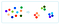Example: RGB are the latent factors for color, and all colors can be expressed via RGB

## Math Model of Exploratory Factor Analysis (EFA)

Exploratory Factor Analysis (EFA) model discovers the linear relationship between the observed training variables(a.k.a feature) and latent factors as below

Here

1. X ( x, x, ⋅⋅⋅ , xₚ ) is observed training set with p features
2. F ( f₁, f, ⋅⋅⋅ , fₖ ) is common factors for data set X ( x, x, ⋅⋅⋅ , xₚ ) and kp, each fᵢ is independent, fᵢ ~N(0,1) and Cov(F) = Iₖ
3. B (β₁, β₂, ⋅⋅⋅ , βₚ ) is stochastic error terms, βᵢ is for i-th feature, each βᵢ is independent and βᵢ ~N(0, σᵢ²)
4. A ( p × k ) is factor loading matrix, αᵢⱼ is factor j weight on feature i, the larger absolute value of αᵢⱼ, the larger impact factor j on feature i

Variable(feature) Variance Analysis

For any feature variable xᵢ in model, calculate its variance

Var(xᵢ)

= αᵢ₁² Var(f₁) + αᵢ₂² Var(f₂) + ⋅⋅⋅ + αᵢₖ² Var(fₖ) + Var(βᵢ)

= Σj αᵢⱼ² + σᵢ² (j = 1,2,⋅⋅⋅,k)

If σᵢ² is zero or very small, then factor loading αᵢⱼ can fully explain the variance of variable xᵢ, so the latent factor combination is valid to represent variable xᵢ. In EFA Σj αᵢⱼ² is called communality.

Factor Variance Analysis

Based on factor loading matrix A(αᵢⱼ)

Factor i loading variance gᵢ² = Σj αⱼᵢ² (j=1,2,⋅⋅⋅,p)

Because factor importance depends on the factor loading variance , so we can use to select the relatively important factors

All factors variance is Σi gᵢ² (i=1,2,⋅⋅⋅,k)

All error terms variance is Σi σᵢ² (j=1,2,⋅⋅⋅,p)

The percentage of variance explained by factors as below , and if this percentage is close to 1, then it prove the valid of the factors

All factors variance / (All factors variance + All error terms variance) =

Σi gᵢ² (i=1,2,⋅⋅⋅,k) / ( Σi gᵢ² (i=1,2,⋅⋅⋅,k) + Σi σᵢ² (j=1,2,⋅⋅⋅,p) )

## Factor Extractions

Factor extraction is to resolve the above EFA math model and computer the factor loading matrix A. There are two popular Factor Extraction ways, one is Principal Component Analysis(PCA), the other is Common Factor Analysis.

Principal Component Analysis can computer the factor loading matrix which can explain the maximum variance of the original dataset, and is used when we need to drive the minimum number of factors and explain the maximum portion of variance in the original dataset

Common Factor Analysis splits the original dataset variance into common variance and unique variance(σᵢ²), and the factor loading only explains the common variance. In practice, Common Factor Analysis is used when we need searching for the latent factors underlying the relationships between training set variables

## Factor Rotation

Factor Rotation is driving factor loading matrix(a.k.a axes) to turn around the origin, when axes turning, the factor loading and variance will change accordingly.

The normal purpose of rotation is to maximizes high factor loadings and minimizes low factor loadings, therefore each variable can only be dominated by fewer high loading factors which make it easier to explain the relationship between original variable and factors. Please check reference 10 for detailed rotation definition and choice.

## Python Example for Exploratory Factor Analysis

The example data is from Kaggle US Airline passenger satisfaction survey. In the survey, there are 14 service quality questions and passengers can score from 0 to 5, so we only keep these 14 columns and try to discover the latent factors underneath the 14 questions.

`#pip install factor_analyzerimport numpy as npimport matplotlib.pyplot as plt import pandas as pd  import seaborn as sns from factor_analyzer import FactorAnalyzer%matplotlib inlinesatis_df= pd.read_csv("satisfaction_v2.csv")satis_df.drop(['id',         'satisfaction_v2',         'Gender',         'Customer Type',         'Type of Travel',         'Class',          'Age',         'Flight Distance',          'Departure Delay in Minutes',          'Arrival Delay in Minutes'        ], axis=1, inplace=True)correlation_matrix = satis_df.corr().round(2)plt.figure(figsize = (16,8))sns.heatmap(data=correlation_matrix, annot=True)`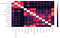Correlation Matrix

Step 1 Assumption Test - Training data need satisfy the below two test to carry on Factor Analysis

`'''Bartlett’s Test is to determine there are correlations in source data variables'''from factor_analyzer.factor_analyzer import calculate_bartlett_sphericitychi_square_value,p_value=calculate_bartlett_sphericity(survey_df)chi_square_value, p_value# (785755.7298083812, 0.0)# p_value = 0.0 indicate no correlation assumption is rejected'''Kaiser-Meyer-Olkin Test is to determine there are adequate data in source dataset to carry on factor analysis'''from factor_analyzer.factor_analyzer import calculate_kmokmo_all,kmo_model=calculate_kmo(survey_df)kmo_model# 0.7946981282043835# kmo_model > 0.6 indicate source data is adequate`

Step 2 Decide how many latent factors as major contribution factors

`fa = FactorAnalyzer(14, rotation=None)fa.fit(survey_df)ev, v = fa.get_eigenvalues()plt.scatter(range(1,survey_df.shape+1),ev)plt.title('Scree Plot')plt.xlabel('Factors')plt.ylabel('Eigenvalue')plt.grid()plt.show()`

Normally FA only take factors whose eigenvalue >1, so in our example we only consider 3 factors

Step 3 Performing Factor Analysis

`'''rotation="varimax" becasuse we want few large and lots of close to 0 loadings'''pd.options.display.float_format = "{:,.3f}".formatfa = FactorAnalyzer(3, rotation="varimax")fa.fit(survey_df)loading_df = pd.DataFrame(data=fa.loadings_, columns=['Factor 1','Factor 2','Factor 3'])loading_df['Feature'] = survey_df.columnscolumn_names = ['Feature','Factor 1','Factor 2','Factor 3']loading_df = loading_df.reindex(columns=column_names)loading_df`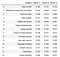Factor Loading

Step 4 Grouping Feature by Factor Loading

In our example, there are only 3 factors, so we use the largest factor to group all feature into 3 groups, as below color indicated

Step 5 Factor Interpretation

Based on grouping features, we can abstract the factor implications

Factor 1 - Technology

Inflight WiFi service, Inflight entertainment, Online support, Ease of Online booking, Online boarding

Factor 2 - Service

On-board service, Leg room service, Baggage handling, Checkin service, Cleanliness

Factor 3 - Convenience

Seat comfort, Departure/Arrival time convenience, Food and Drink, Gate Location

Step 6 Factors as Feature for downstream modelling

From factor loading matrix, we can reverse the linear relationship and use original variables to calculate the factor values and use factor values as model features.

In our example, the original 14 correlated features are reduced to 3 uncorrelated factor features

`fa = FactorAnalyzer(3, rotation="varimax")factor_feature = fa.fit_transform(survey_df)factor_feature_df = pd.DataFrame(    data=factor_feature,     columns=['Technology','Service','Convenience'])factor_feature_df`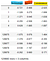Factor Features

# REFERENCE

Data Scientist & Engineer from Sydney

## More from Summer Hu

Data Scientist & Engineer from Sydney